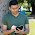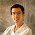## Tuesday, August 07, 2007

### Is Pi defined in the best way?

&pi, the ratio of the circumference to the diameter of a circle, is one of the most important constants in Math. However, &pi could just as easily have been defined as the ratio of the circumference to the radius of a circle. This would not change math in any serious way, but it would make some formulas simpler. Think about how often `2*&pi' comes up in formulas.

This theme was explored by Bob Palais in this article. He makes a good case. I look at two examples not in the article, one of which supports his case, and the other is a matter of taste. During this blog I will denote the ratio of Circumference to Radius by PII.

EXAMPLE ONE: Consider the volume and surface area of an n-dim sphere. There is no closed form formula (that I know of) but there is a recursive formula. See this. The following table shows, for each n, the volume of an n-dim sphere divided by Rn.
 n Trad Vol/Rn New Vol/n 1 2 2 2 &pi (1/4)*PII 3 (4/3)*&pi (1/6)*PII 4 (1/2)*&pi2 (1/32)*PII2 5 (8/15)*&pi2 (1/60)*PII2 6 (1/6)*&pi3 (1/382)*PII3 7 (16/105)*&pi3 (1/1640)*PII3
Is the New Volume easier or harder? A little easier in that all of the numerators are 1. But no real pattern. Similar is true for surface area. Are these formulas better? That is a matter of taste.

EXAMPLE TWO: The Zeta Function is

&zeta(n) = &sum r-n (The sum is from r=1 to infinity.)

It is known that

&zeta(2n) = (-1)n-1 ((2*&pi)2n/2(2n)!)B2n

where Bn is the nth Bernoulli Number. If we use PII instead we get the simpler

&zeta(2n) = (-1)n-1 ((PII)2n/2(2n)!)B2n

This is BETTER!

1.> There is no closed form formula (that I know of)

n even: V(2n) = pi^n / n! for n
n odd: V(2n+1) = pi^n 2^(2n+1) n! / (2n+1)!

2.There are some genuine points that he makes. But the issue is at worst a minor pedagogical inconvenience and gets way more attention than it deserves. I've seen it brought up several times already in blogs and magazines.

3.Actually V(n) = pi^(n/2)/(n/2)! for all n, even and odd. You should use the Gamma function to interpret non-integral factorials. (And just as pi itself is mistakenly half of what it should be, the Gamma function is mistakenly shifted by one.)

4.limited pi, or

Pi, 3.1415676 equals 19 divided by 6.047936

5.Currently e to the power pi times i equals minus one. I would hate it if this equation would need mending.

>>>> JL Balcázar

6.It may be that 2*pi*i is an even more fundamental constant than 2*pi or pi. It is, after all, the generator of log(1). The fact that so many formulae involving pi^n depend on the parity of n is another clue in this regard.

1.Even, in fact, pi is (proved)"wrong" (according to one side)... A still believes that mathematics is not just as an agreement of symbols, but more about agreement of ideas ... So let it be as "evolutional" symbols and ideas. We love pi as we love mathematics as historical-evolutional product... I

7.Jose' (greetings!), I've thought about your point as a reason to keep the original definition of pi. But the revised version also gives a sense of perfection and completeness. Compare:

e^{\pi*i} + 1 = 0

uses each of {0,1,e,\pi,i,=,+,*,^} exactly once. But

e^{\PII*i/2} + 1 = 0

throws in 2 and division without duplicating anything. Subtraction is still missing, but (IMHO) division really has more of a separate character from multiplication as a fundamental mathematical operation, than subtraction has from addition.

Ah, I see Palais touches on this in his article.

8.Another equation for limited pi comes from Nicomachus

2.714258714258714258 = 57/21

and that's 19 digits. According to numbers that leaves three numbers out of 9...369.

9.pi = 3.14159265

10.Google doesn't know everything, and neither do I, but I know you can find a perfect circle by following the pattern to limited pi, and it's simple.

Magic squares, although it's not a magick trick.

11.Is the upside down Pi symbol used for anything else? If not just use it to denote the ratio of circumference to radius.

12.Sometimes the 2*pi combination appears often enough that people do redefine it. In physics h/(2*pi) appears so many times, it is redefined as hbar ---an 'h' with a slash through it ($\hbar$ in LaTeX). h is of course, Plank's constant.

13.A lemma in a paper I'm writing deals with the distortion between the circular distance angle(x,y) and the straight-line distance |x - y| between two points x,y on the unit circle. The maximum distortion, for antipodal points, is

\pi/2.

Not only is this fundamental, but one can contend that the distortion angle(x,y)/|x - y| is the first aspect of circular measurement that concerned the ancients.

Hence I am agreed that the "true" value of "pi" is off by a factor of 2---but in the opposite direction! ;->

14.With this new constant

e^(i * PII) = 1

However, pi does appear on it's own in a *lot* of equations. You're just picking the ones where it is 2pi and saying "ooo look it would be easier".

It's easier to write n * pi than pi/n in equations so I'd say they made the right decision. If you really can't stand the 2 in '2 pi r' you can use 'pi d' (diameter) instead.

Enough of this nonsense.

15.Regarding the original page on Pi being wrong (http://www.math.utah.edu/~palais/pi.html), and the author's observations at the bottom of the page regarding the area and circumference of a circle:

"A couple of my own observations since the article. It seems to me that you can't have it both ways on area A= πr2 and circumference C= πd. If you believe diameter is fundamental, then it should be A= πd2/4."

I would tend to think that radius is more fundamental than diameter (so circumference = (turn)r, clearly more elegant than 2πr ), but think for a minute about the area formula: area = π r^2. A circle, if you think about it, is effectively a triangle that has been wrapped around the origin; its point is at the origin, and its base (size 2π) wraps around the circumference. Since the formula for a triangle is 1/2 base * height, it's only reasonable that the formula for the area of a circle is 1/2 (turn) r. Similarly, since the surface area of a 3-sphere is 2(turn)r^2, the volume of a 3-sphere is logically (1/3) * 2(turn)r^3, considering that the volume of a pyramid is (1/3) base * height. The surface area of a 4-sphere is (1/2)(turn^2)r^4, so the volume is (1/4)(1/2)(turn^2)r^4. Incidentally, the table of volumes shown above is incorrect: the sequence for New Vol should be:

2,
(1/4)*(turn),
(2/3)*(turn),
(1/8)*(turn^2),
(2/15)*(turn^2),
(1/48)*(turn^3),
(2/105)*(turn^3),

which looks a lot nicer than the traditional list, because it clearly shows the parity dependence of the formula with alternating 1's and 2's in the numerator.

Fascinating article!

16.Whoops, the second term under New Vol should be (1/2)(turn), of course, not (1/4)(turn).

17."Currently e to the power pi times i equals minus one. I would hate it if this equation would need mending."
>>>> JL Balcázar

Apart from the suggestion by KWRegan, what about:

e ^ (i * PII) + 0 = 1

That still incorporates the fundamental constants (0; 1; e; i; PII) and the fundamental operations (addition; multiplication; exponentiation).
- :)

18.:50 PM, August 07, 2007
> There is no closed form formula (that I know of)

n even: V(2n) = pi^n / n! for n
n odd: V(2n+1) = pi^n 2^(2n+1) n! / (2n+1)!

19.Let tau = 2pi
Let C = tau r = a "circumference" of an n-sphere
Let A(n) = "surface" of an n-sphere
Let V(n) = "volume" of an n-sphere
Basis:
Let V(0) = 1 = a single point in 0-dim space
Let A(1) = 2 = points a given r from a center point in 1-dim space
For all n in Natural:
V(n) = A(n)(r / n)
A(n) = V(n-2) C = V(n-2) (tau r)

Thus:
Even dimensions:
A(0) = undefined
V(0) = 1
A(2) = tau r
V(2) = (1/2) tau r^2
A(4) = (1/2) tau^2 r^3
V(4) = (1/2*4) tau^2 r^4
A(6) = (1/2*4) tau^3 r^5
V(6) = (1/2*4*6) tau^3 r^6
A(8) = (1/2*4*6) tau^4 r^7
V(8) = (1/2*4*6*8) tau^5 r^8
....

Odd dimensions:
A(1) = 2
V(1) = 2r
A(3) = 2 tau r^2
V(3) = (2/3) tau r^3
A(5) = (2/3) tau^2 r^4
V(5) = (2/3*5) tau^2 r^5
A(7) = (2/3*5) tau^3 r^6
V(7) = (2/3*5*7) tau^3 r^7
A(9) = (2/3*5*7) tau^4 r^8
V(9) = (2/3*5*7*9) tau^4 r^9
...

Hence:
V(n) = (tau^(floor(n/2)) r^n / n!!)(n mod 2 + 1)
A(n) = (tau^(floor(n/2)) r^(n-1) / (n-2)!!)(n mod 2 + 1)

You can only see this unification over all dimensions when you cast these formulas in terms of tau. If you substitute 2pi, and then "exploit" those factors of 2 to cancel out factors in the even dimensions, but leave them as clutter in the odd dimensions, you DESTROY this unification.

pi has no justification as the "circle" constant, because it only represents _half_ a circle.